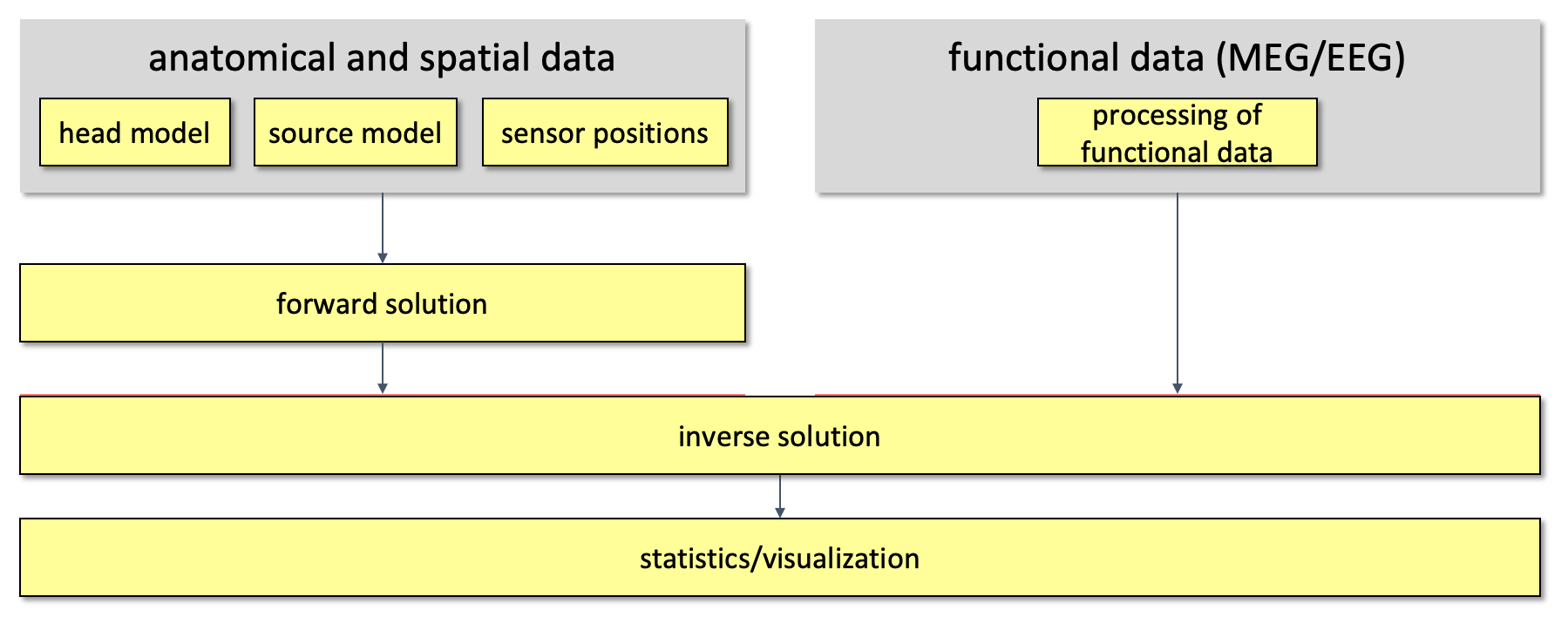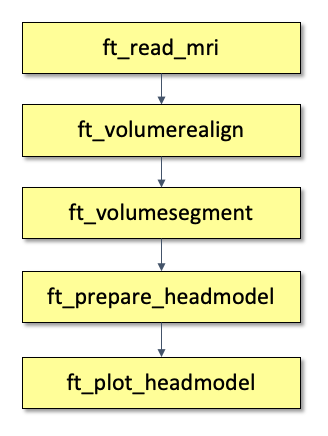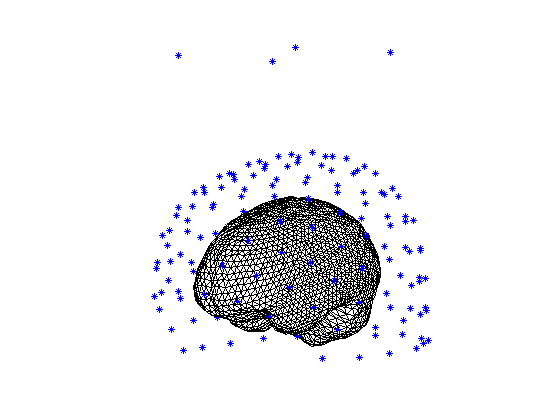# Creating a volume conduction model of the head for source reconstruction of MEG data

## Introduction

This tutorial describes how to construct a volume conduction model of the head (head model) based on an individual subject’s MRI. We will use the anatomical images that belong to the same subject whose data were analyzed in the Preprocessing - Segmenting and reading trial-based EEG and MEG data and the Event-related averaging and MEG planar gradient tutorials. The corresponding anatomical MRI data is available from the download server.

The volume conduction model of the head that will be constructed here is specific to the computation and source reconstruction of MEG data. Different strategies can be used for the construction of head models. The processing pipeline of the tutorial is an example which we think is the most appropriate for the tutorial-dataset.

This tutorial will not show how to perform the source reconstruction itself. If you are interested in source reconstruction methods, you can go to the Localizing oscillatory sources using beamformer techniques and to the Source reconstruction of event-related fields using minimum-norm estimate tutorials.

The volume conduction model created here is MEG specific and cannot be used for EEG source reconstruction. If you are interested in EEG source reconstruction methods, you can go to the corresponding EEG tutorial.

## Background

The EEG/MEG signals measured on or around the scalp do not directly reflect the activated neurons in the brain. To reconstruct the actual activity in the brain, source reconstruction techniques are used. You can read more about the different methods in the review papers that are listed here.

The activity in the brain is estimated from the EEG or MEG signals using

1. the EEG/MEG activity itself that is measured on or around the scalp
2. the spatial arrangement of the electrodes/gradiometers relative to the brain (sensor positions),
4. the location of the source (source model)

Using this information, source estimation comprises two major steps: (1) Estimation of the EEG potential or MEG field distribution for a known source is referred to as forward modeling. (2) Estimation of the unknown sources corresponding to the measured EEG or MEG is referred to as inverse modeling.

The forward solution can be computed when the head model, the sensor positions and a model for the source are given. For distributed source models and for scanning approaches such as beamforming, the source model consists of a discrete description of the the brain volume or of the cortical sheet in many voxels or vertices. When the forward solution is computed, the lead field matrix (with dimensions Nchan * Nsources) is calculated for each point, taking into account the head model and the sensor positions.

A prerequisite of forward modeling is that the geometrical description of the sensor positions, head model and source model are expressed in the same coordinate system (e.g., CTF, MNI, Talairach) and with the same units (mm, cm, or m). There are different conventions for coordinate systems. The precise coordinate system is not relevant, as long as all data is consistent. Here you can read how the different head and MRI coordinate systems are defined. For most MEG systems, the gradiometers are by default defined relative to head localizer coils or anatomical landmarks, therefore when the anatomical MRI are aligned to the same landmarks, the position of the MEG sensors directly matches the MRI. As EEG data is typically not explicitly aligned relatively to the head, therefore, the EEG electrodes usually have to be explicitly realigned prior to source reconstruction (see also this faq and this example).Figure 1. Overall outline of the pipeline used for source reconstruction

This tutorial is focusing on how to build the volume conduction model for the head.

A volume conduction model of the head, also known as a head model, is represented in FieldTrip as a MATLAB structure. It describes how the currents flow through the tissue, not where they originate from. In general it consists of a description of the geometry of the tissue(s), a description of the conductivity of the tissue(s), and mathematical parameters that are derived from these. How the mathematical parameters are described depends on the computational solution to the forward problem, either by numerical approximations (e.g., using the boundary element and finite element method, or BEM and FEM), or by exact analytical solutions (e.g., for spherical models).

The more accurate the description of the geometry of the head or the source, the better the quality of the forward model. There are many types of head models which, to various degrees, take the individual anatomy into account. The different head models available in FieldTrip are listed here.

In this specific tutorial we will use a semi-realistic head model developed by Nolte (2003) that assumes a realistic information about the interface between the brain and the skull. This outer brain surface will be extracted from the anatomical MRI images of the subject. First, we will use anatomical MRI of the subject to extract the brain surface from the anatomical images, which is termed segmentation. Note that the segmentation procedure is quite time consuming. Following the segmentation of the anatomical images, a description of the surface using vertices and triangles is constructed. Finally, the single-shell head model will be computed.

If an anatomical MRI is not available for your MEG subject, you can consider to use a template MRI or a template head model that is located in the FieldTrip template directory. If you do not have an MRI, but if you do have a measurement of the scalp surface (e.g., with a Polhemus tracker), you can use a local spheres volume conduction model. If you do not want to (or cannot) use any realistic information about the brain-surface or the head-shape, you can resort to the single sphere volume conduction model.

## Procedure

We will create a head model based on the anatomical mri of the tutorial data set which is available here. The pipeline is depicted in Figure 2.Figure 2. Pipeline of creating and visualizing a head model

### Reading in the anatomical data

Before starting to use FieldTrip, it is important that you set up your MATLAB path properly. You can read about how to set up your MATLAB path here.

``````cd <path_to_fieldtrip>
ft_defaults
``````

Then, you can read in the mri data.

``````mri = ft_read_mri('Subject01.mri');

disp(mri)
dim: [256 256 256]
anatomy: [256x256x256 int16]
hdr: [1x1 struct]
transform: [4x4 double]
unit: 'mm'
coordsys: 'ctf'
``````

The structure of your mri variable contains the following field

• dim: This field gives information on the size (i.e. the number of voxels) of the anatomical volume into each direction.
• anatomy: This is a matrix (with the size and number of dimensions specified in dim) that contains the anatomical information represented by numbers.
• hdr: Header information of the anatomical images.
• transform: A transformation matrix that aligns the anatomical data (in field anatomy) to a certain coordinate system.
• coordsys: The description of the coordinate system which the anatomical data is aligned to.

You can see that the coordsys field of anatomical data that we read in is already aligned to the ctf coordinate system. This can be done using the CTF specific MRIConverter and MRIViewer software as outlined here or using the ft_volumerealign function.

It is also possible to read in anatomical MRI data in other formats, which are defined in a different coordinate system. If your anatomical MRI is not aligned to the ctf coordinate system, it can be aligned using ft_volumerealign function. For this, you will need to align your MRI to the fiducial points.

When you read in your own anatomical data, it may not give information on the coordinate system in which the anatomical data is expressed and/or maybe there is no transformation matrix specified. In this case, you can check the coordinate-system with the ft_determine_coordsys function.

### Segmentation

In this step, the voxels of the anatomical MRI are segmented (i.e. separated) into different tissue types . By default, the gray matter, white matter and the cerebro-spinal fluid (csf) compartments are differentiated. Based on these compartments a so called brainmask is created, which is a binary mask of the content inside the skull. All voxels that are inside the skull (i.e. the complete brain) are represented by 1, all other voxels by 0. The function ft_volumesegment will produce the required output.

Note that the segmentation is quite time consuming and if you want you can load the result and skip ahead to the next step. You can download the segmented MRI of this tutorial data from the download server (segmentedmri.mat).

``````cfg           = [];
cfg.output    = 'brain';
segmentedmri  = ft_volumesegment(cfg, mri);

save segmentedmri segmentedmri

disp(segmentedmri)
dim: [256 256 256]
transform: [4x4 double]
coordsys: 'ctf'
unit: 'mm'
brain: [256x256x256 logical]
cfg: [1x1 struct]
``````

The segmentedmri data structure contains the following field

• dim

• transform

• coordsys

• unit: unit of measurement of the voxels

• cfg: configuration information of the function which created segmentedmri

The segmentation does not change the coordinate system, nor the size of the volume. You can see this in the first three fields (dim, transform and coordsys) which are the same as the corresponding fields of the input mri data structure. But now, the field transform aligns the matrix in field brain (which contains the brainmask) to the coordinate system defined in the coordsys field.

Alternatively, you can also leave out the definition of the cfg.output. In this case, the function will output the default segmentation that are the probabilistic values of the gray, white and csf compartments. In this case, the brain mask will be automatically created in the next step by the ft_prepare_headmodel function. For further information on the different segmentation options, read the help of ft_volumesegment.

Once the brain mask is segmented out of the anatomical MRI, a surface description of the brain is constructed and the volume conduction model . We will specify method ‘singleshell’ to build the head model in the cfg.method field using ft_prepare_headmodel.

``````cfg = [];
cfg.method='singleshell';

save vol vol

disp(vol)
bnd: [1x1 struct]
type: 'singleshell'
unit: 'mm'
cfg: [1x1 struct]
``````

The vol data structure contains the following field

• bnd: contains the geometrical description of the head model.

• type: describes the method that was used to create the headmodel.

• unit: the unit of measurement of the geometrical data in the bnd field

• cfg: configuration of the function that was used to create vol

The bnd field describes a surface with vertices and triangles (in the bnd.pnt and bnd.tri fields) as the geometrical description of the volume conductor.

This tutorial does not intend to make a elaborative comparison of the different volume conduction models, nor to discuss their relative merits.

The method used in this tutorial is based on Nolte G. (2003) The magnetic lead field theorem in the quasi-static approximation and its use for magnetoencephalography forward calculation in realistic volume conductors. We recommend this method for most general MEG situations.

The paper Lalancette M, Quraan M, Cheyne D. (2011) Evaluation of multiple-sphere head models for MEG source localization discusses another popular method for MEG forward modeling, which is based on fitting local spheres to the surface. This alternative method can be used by specifying ‘localspheres’ as method in ft_prepare_headmodel.

Alternatively, you can also create and use a multiple-layered head model with Openmeeg. For this you can follow the procedure described in the tutorial for creating a volume conduction model for EEG data

### Visualization

The head model contains the brain-skull boundary as the geometrical description of the head. You can visualize this using the following code. First, we will plot the sensors (MEG channels) with the ft_plot_sens function. Second, we will plot the head model with ft_plot_headmodel in the same figure with the sensors. In order to plot also the location of the MEG channels, we read in the location of the channels using the .ds file from the tutorial data and the ft_read_sens function. The Subject01.zip MEG dataset includes the MEG data and the anatomical MRI. The units of the headmodel are defined in ‘mm’, while the units of the sensors are in ‘cm’. When we plot the headmodel together with the sensors, they need to have the same units. Therefore, the units of the headmodel will be converted to ‘cm’ with the ft_convert_units function.

``````vol = ft_convert_units(vol, 'cm');

figure
ft_plot_sens(sens, 'style', '*b');

hold on
``````Figure 3. The geometry of the volume conduction model of the head using method “singleshell”

When the figure is plotted, you can look at the figure from different views using the curved arrow in the MATLAB figure menu. Note that there are 4 channels hovering above the normal channels; those are the MEG reference channels that can be used for environmental noise suppression.

### Exercise 1

Create a head model with method ‘singlesphere’ that you fit on the inside brain surface, i.e. using the output of the already made segmentation.

Plot both head models in the same figure, check the help of ft_plot_headmodel for further options of the visualization (e.g., color, transparency) which help to see the two head models together.

What is the difference between the head models?

### Exercise 2

In exercise 1, you created a head model with method ‘singlesphere’. How is its geometrical description defined? What is the difference between the fields of the single sphere and single-shell model which contain the geometrical description?